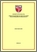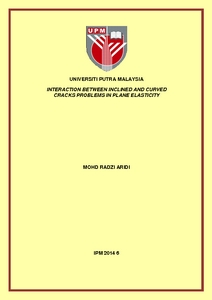Simple Search:

Interaction between inclined and curved cracks problems in plane elasticity

Citation

Aridi, Mohd Radzi (2014) Interaction between inclined and curved cracks problems in plane elasticity. Masters thesis, Universiti Putra Malaysia.

Abstract / Synopsis

In this thesis, the interaction between inclined and curved cracks problem in plane elasticity is formulated into the hypersingular integral equations using the complex variable function method. Then, using the curved length coordinate method, the cracks are mapped into a straight line which require less collocation points, hence give faster convergence. In order to solve the equations numerically, the quadrature rules are applied and we obtained the unknown coeficients with M+1 collocation points. The obtained unknown coeficients will later be used in calculating the stress intensity factors. Firstly, we investigated the problems between straight and curved cracks problem in plane elasticity. The results give good agreements with the existence results. Then, we extended the problem for the interaction between inclined and curved cracks problem in plane elasitcity. For numerical examples, four types of loading modes have been presented : Mode I, Mode II, Mode III and Mix Mode.Preview
PDF
IPM 2014 6RR.pdfView Item